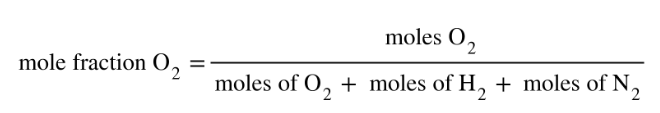# Problem: What is the mole fraction of O2 in a mixture of 15.1 g of O2, 8.18 g of N2, and 2.47 g of H2?

###### FREE Expert Solution
• Mole fraction can be calculated as:• Calculate for the moles of each gas using the given mass and their MM. Mole fraction of O2 will appear as:88% (209 ratings)###### Problem Details

What is the mole fraction of O2 in a mixture of 15.1 g of O2, 8.18 g of N2, and 2.47 g of H2?